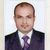# QlikView App Dev

Discussion Board for collaboration related to QlikView App Development.

Announcements
QlikWorld online is next week! REGISTER NOW
cancel
Showing results for
Did you mean:Creator

## Avoid counting duplicate values in straight table

Hi all,

I have a straight table where it shows duplicate values. I would like to count the ordered quantity only once for each order. I used expression sum(Ordered Quantity) for Ordered Quantity and it is counting the duplicate values too.

In the below example, order 15562 has three distinct block codes and hence shows the ordered quantity thrice. So, when I use the expression sum([Ordered Quantity]) it shows sum as 3 whereas we have only one ordered quantity for that order. How can I make the Ordered quantity to show 1 for the below order. Any ideas?Thanks!

1 Solution

Accepted SolutionsMVP

More like this

Sum(Aggr([Ordered Quantity], [Order Number]))

or

Sum(Aggr(Only([Ordered Quantity]), [Order Number]))

or

Sum(Aggr(Sum(DISTINCT [Ordered Quantity]), [Order Number]))

or

Sum(Aggr(Avg([Ordered Quantity]), [Order Number]))

6 RepliesMVP

May be this?

Sum(Aggr(sum([Ordered Quantity]), [Order Number]))

Before develop something, think If placed (The Right information | To the right people | At the Right time | In the Right place | With the Right context)MVP

More like this

Sum(Aggr([Ordered Quantity], [Order Number]))

or

Sum(Aggr(Only([Ordered Quantity]), [Order Number]))

or

Sum(Aggr(Sum(DISTINCT [Ordered Quantity]), [Order Number]))

or

Sum(Aggr(Avg([Ordered Quantity]), [Order Number]))Creator
Author

Thanks to both sides of you. Sunny's expression did the trick for meMaster

Hi Sangeetha,

If you still want to show your value in a straight table then maybe:

Order Date Order Number Order Line Block Code Sum(Aggr(only([Ordered Quantity]), [Order Number],[Order Line]))
3
09/05/201715592190MISC1
09/05/201715592190PRICE0
09/05/201715592190SHIP TO0
09/05/201715592191MISC1
09/05/201715592191PRICE0
09/05/201715592191SHIP TO0
09/05/201715593190MISC1
09/05/201715593190PRICE0
09/05/201715593190SHIP TO0

I added a line to your order and a new order too to show the effect of more data.

Regards

AndrewMVP

You have different order dates and different Block code also for the Quantity so it is shown 1 and total should be 3  for each quantity where else try some of this

Sum(Aggr(Sum(DISTINCT [Ordered Quantity]), [Order Number]))

Or

Count(Aggr(Sum(DISTINCT [Ordered Quantity]), [Order Number]))Contributor III

I dont sure to understand, have you think to use also dimensionality() function as possibility to differentiate total in different lier of data?